## Example Questions

### Example Question #15 : Polynomials

Simplify the following expression.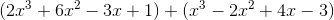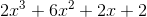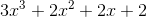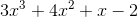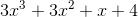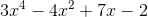Explanation:

Line up each expression vertically. Then combine like terms to solve.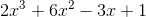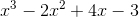____________________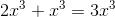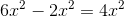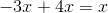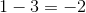Thus, the final solution is.

### Example Question #16 : Polynomials

What is the value ofwhen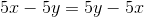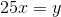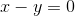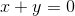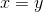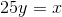Explanation:In adding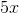to both sides: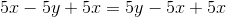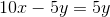. . .and adding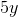to both sides: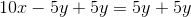. . .the variables are isolated to become: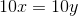After dividing both sides by, the equation becomes:### Example Question #17 : Polynomials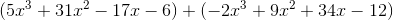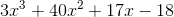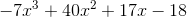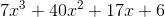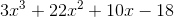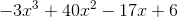Explanation:

This is a problem where elimination can be help you save a little time. You can eliminate options quickly by simplifying one power at a time and comparing your work with the answer choices.

To begin, reorder the problem so that all like terms are next to each other. When doing so, keep an eye on your signs so that you don't accidentally make a mistake.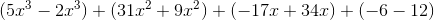From here, combine each pair of terms. As you do so, compare your work with the answer choices.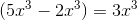Eliminate any answer choices that have a different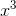term.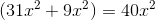Eliminate any answer choices that have a differentterm.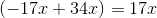Eliminate any answer choices that have a different x term.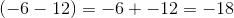Eliminate any answer choices that have a different constant term.

Once you put all of your solutions together, the correct answer looks like this: